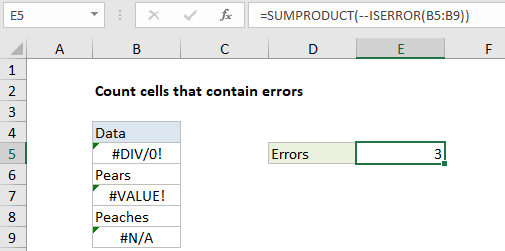## Excel Office

Excel How Tos, Tutorials, Tips & Tricks, Shortcuts

# Count cells that contain errors in Excel

This tutorial shows how to Count cells that contain errors in Excel using the example below;

## Formula

=SUMPRODUCT(–ISERR(range))## Explanation

To count cells that contain errors, you can use the ISERR function, wrapped in the SUMPRODUCT function.  In the example shown, E5 cell contains this formula:

`=SUMPRODUCT(--ISERROR(B5:B9))`

### How this formula works

SUMPRODUCT accepts one or more arrays, multiplies the arrays together, and returns the “sum of products” as a final result. If only one array is supplied, SUMPRODUCT simply returns the sum of items in the array.

Worked Example:   Sum columns based on adjacent criteria in Excel

The ISERROR function returns TRUE when a cell contains an error, and FALSE if not. In the example, ISERROR receives a range of cells (B5:B9) as input. Because there are five cells in the range, ISERROR evaluates each cell and returns five results in an array of TRUE / FALSE values:

`{TRUE;FALSE;TRUE;FALSE;FALSE}`

To coerce the TRUE/FALSE values to 1’s and 0’s, we use a double negative (called a double unary). The resulting array looks like this:

`{1;0;1;0;0}`

Finally, SUMPRODUCT sums the items in this array and returns the total, which is 3 in this case.

Worked Example:   Check if range contains a value not in another range in Excel

Note: ISERROR counts all errors. If for some reason you want to count all errors except #N/A, you can use the ISERR function instead.

Worked Example:   Count cells that contain numbers in Excel

### Array formula with SUM

You can also use the SUM function to count errors, but you must enter as an array formula using control + shift + enter. Once entered the formula will look like this:

`{=SUM(--ISERROR(range))}`

The curly brackets are added automatically by Excel and indicate an array formula.